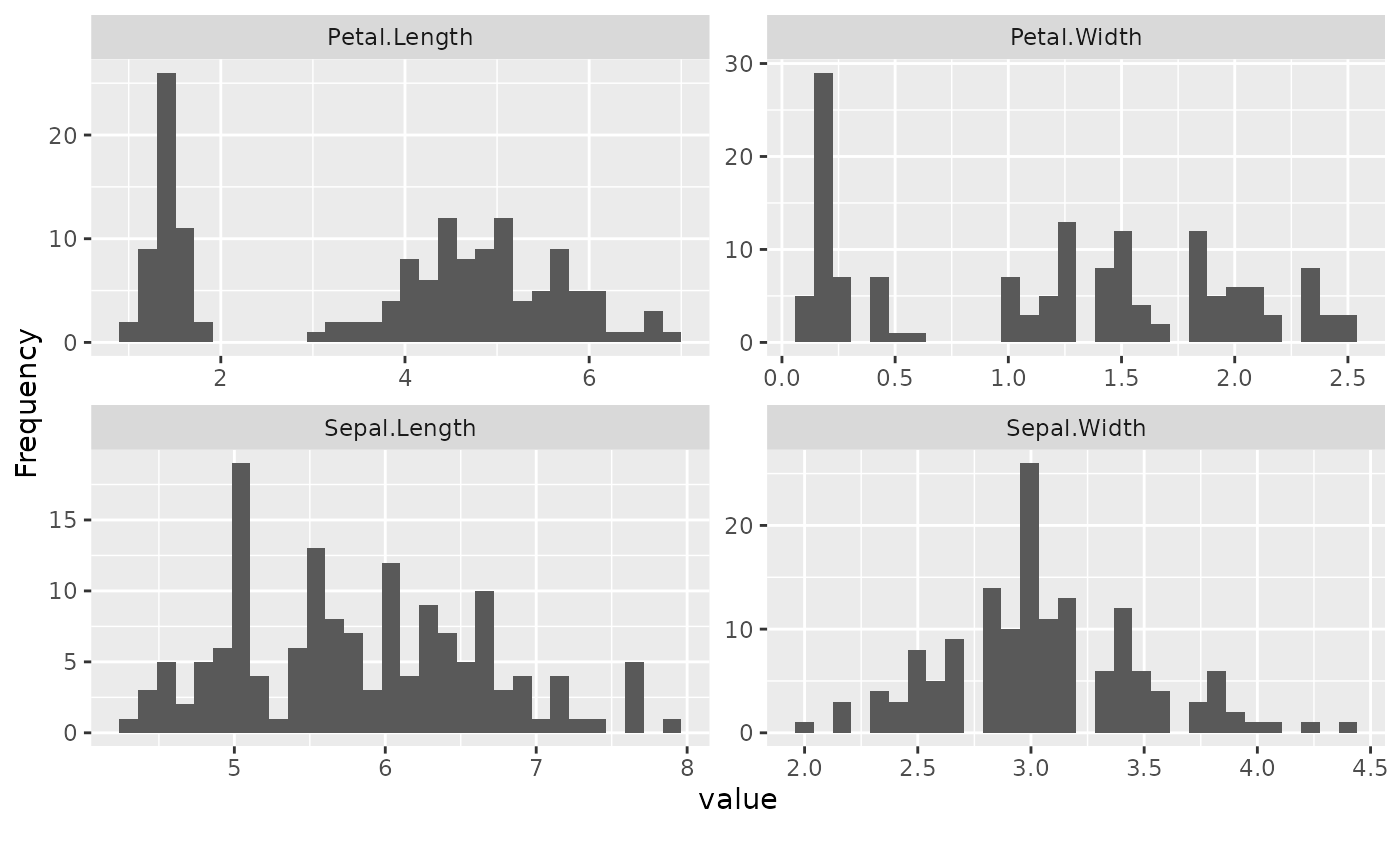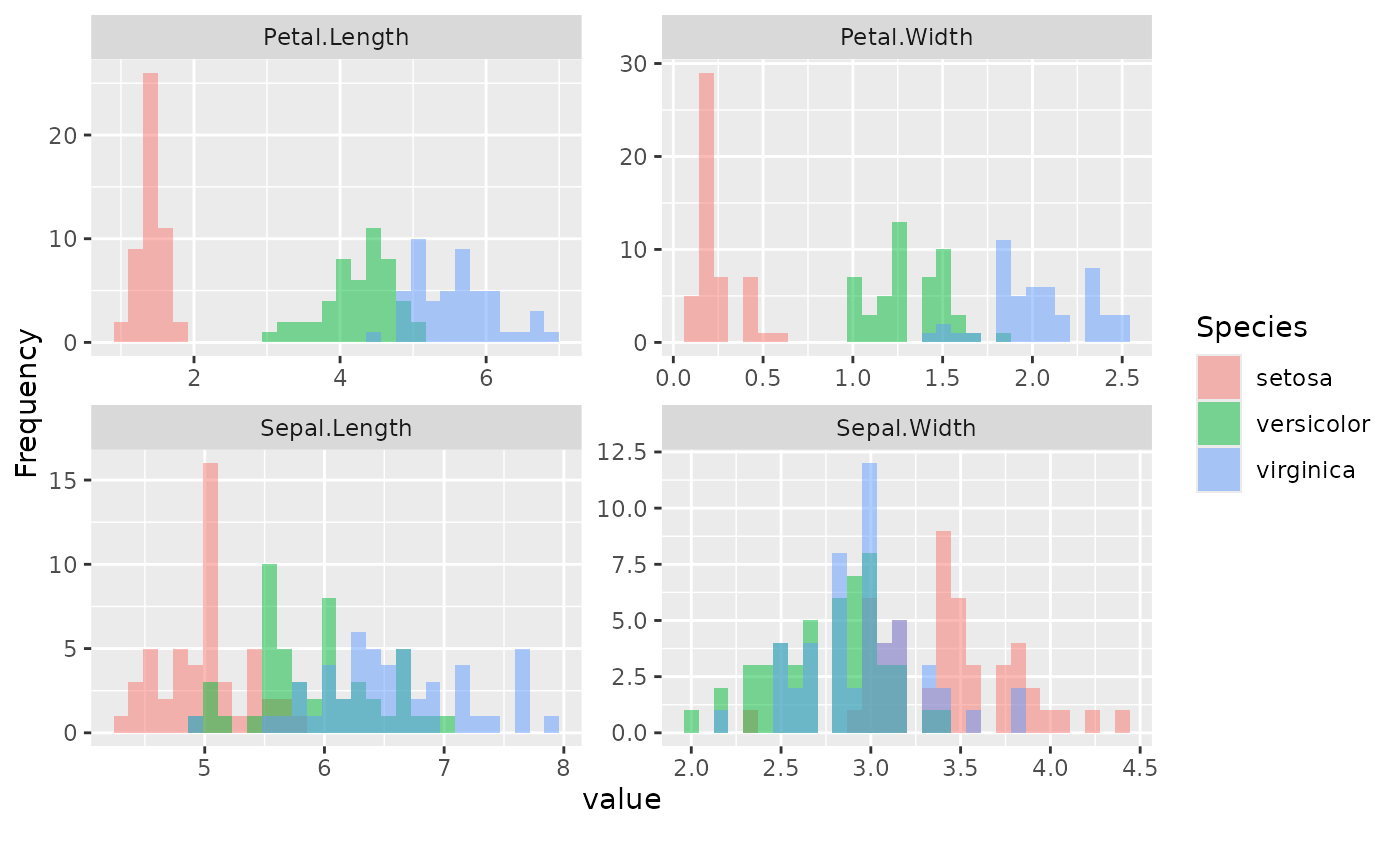Plot histogram for each continuous feature

plot_histogram(data, binary_as_factor = TRUE,
geom_histogram_args = list(bins = 30L), title = NULL,
ggtheme = theme_gray(), theme_config = list(), nrow = 4L,
ncol = 4L, parallel = FALSE)

## Arguments

data input data treat binary as categorical? Default is TRUE. a list of other arguments to geom_histogram plot title complete ggplot2 themes. The default is theme_gray. a list of configurations to be passed to theme. number of rows per page. Default is 4. number of columns per page. Default is 4. enable parallel? Default is FALSE.

## Value

invisibly return the named list of ggplot objects

# Plot iris data
plot_histogram(iris, nrow = 2L, ncol = 2L)plot_histogram(data)